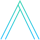# Information Theory with Monica from Friends

August 09, 2019I put Netflix on while I wrote this. Can’t remember what show I was watching though… 🤔

Information theory sits comfortably in between electrical engineering and statistics. Monica sits comfortably in between Ross and Rachel—so naturally, she’s an excellent companion for exploring Claude Shannon’s 1948 masters thesis.

Before we jump in, let’s define information.

Information is the answer to a some question. It is the resolution of an uncertainty.

So, 1 bit of information is the answer to 1 yes-or-no question.

Imagine you and Monica are sitting in the coffee shop, and you say something like:

“Donald Trump tweeted something controversial this morning” - you.

This would be Monica:That’s because Monica gained very little information from your statement. Certainty gives no information, and highly probable events only give a little.

However, if you were to say something like:

“They’ve found intelligent life on Mars.” - you.

Then her reaction would be a bit more like:She just gained a lot of information.

This is a key insight of information theory. The information gained by an event is inversely proportional to it’s probability.

The information gained by an event is inversely proportional to it’s probability.

## Entropy

Entropy is a measure of uncertainty of some message source. It’s given by the following formula:

$H = -\sum p_i \cdot log(p_i)$

Don’t worry, Monica’s gonna break it down for you.The $log(p_i)$ part is the information gained from the response $i$ being given, and $p_i$ is the probability of that response.

Entropy is an expected value, it’s an expected value of information acquired from each symbol sent.

Note - it’s negative because log base 2 of any number in $[0, 1]$ yields a negative, and we want the end result to be positive (since we gain information). More on the negative later.

Imagine you and Monica are sending each other probabilistic messages, where each bit sent is given by a fair coin toss. For a heads, we send a 1; for a tails, we send a 0.

Therefore, a 0 and 1 each have a probability of $\frac{1}{2}$. The entropy of this message source is then given by

\begin{aligned} H &= - (p_0 \cdot log(p_0) + p_1 \cdot log(p_1))\\ &= -(\frac{1}{2} \cdot log(\frac{1}{2}) + \frac{1}{2} \cdot log(\frac{1}{2}))\\ &= -(- \frac{1}{2} - \frac{1}{2})\\ &= 1 \frac{bit}{symbol} \end{aligned}

This means that, on average, it will take 1 bit to send each symbol, or, for every heads or tails response we want to send, it will take one bit.The above table can help give us an idea of some of the values in question. Notice how $log(p)$ is negative—this is why there’s a negative in our entropy formula.

Now, recall that entropy is a measure of uncertainty. Probability matters, since probable events are less informative than improbable ones. If we considered the same scenario, but with an unfair coin, say with a $p_0 = \frac{1}{8}$ (and thus $p_1 = \frac{7}{8}$), then the entropy is:

\begin{aligned} H &= -(p_0 \cdot log(p_0) + p_1 \cdot log(p_1))\\ &= -(\frac{1}{8} \cdot log(\frac{1}{8}) + \frac{7}{8} \cdot log(\frac{7}{8}))\\ &= - (- .2599\ -\ .1168)\\ &= .3678 \frac{bit}{symbol} \end{aligned}

This is a less uncertain message source, so entropy is lower.

Notice how the information yielded by the improbable event, represented by $log(\frac{1}{8})$, is substantially higher than the information yielded by the probable event, represented by $log(\frac{7}{8})$.

Entropy is a measure of uncertainty of a message source.## Encoding Information

This has repercussions on how we can encode information, to send it across a wire (which we do, all the time Monica). We want the message to get there as fast as possible, so the less electric pulses we’re sending through that wire, the better.

The main lesson of the above is the following:

When encoding information, choose the shortest symbols to map to the most probable events, and the longest symbols to represent the least probable ones.

For example, if we were encoding the English language, we could map 1 to “the”, and 1111 to “x”.

This is the essence of a Huffman coding, a very efficient way to encode information.

## Maximizing Entropy

Entropy is maximized when we have equally likely events.

That is because that’s exactly the situation with maximized uncertainty. Note that the entropy formula simplifies a bit for this case ($p_1 = p_2 = ... = p_n = \frac{1}{n}$).

\begin{aligned} H &= -(p_1 \cdot log(p_1) + ... + p_n \cdot log(p_n))\\ &= - (\frac{1}{n} + ... + \frac{1}{n})(log(\frac{1}{n}))\\ &= -\ log(\frac{1}{n}) \end{aligned}

## Final Thoughts

I should probably add that there entropy in information theory and entropy in physics are not exactly the same thing. There is a relationship, but it is not super straightforward. You can read more about it here (and may the force be with you).

In summary, information is a response to a question, and a bit is a response to a yes-or-no question. Entropy is a measure of uncertainty contained within a certain amount of information.

Monica commends you for getting this far.## Sources

1. An Introduction to Information Theory
2. Friends

# Join the mailing list

Get the latest posts in your inbox. No spam, ever.